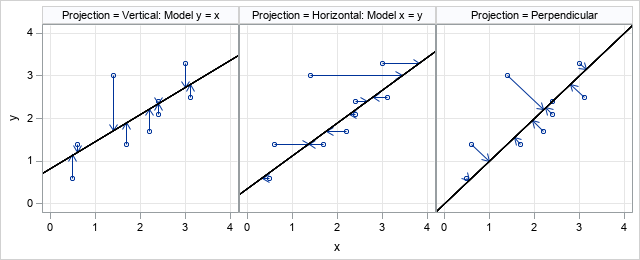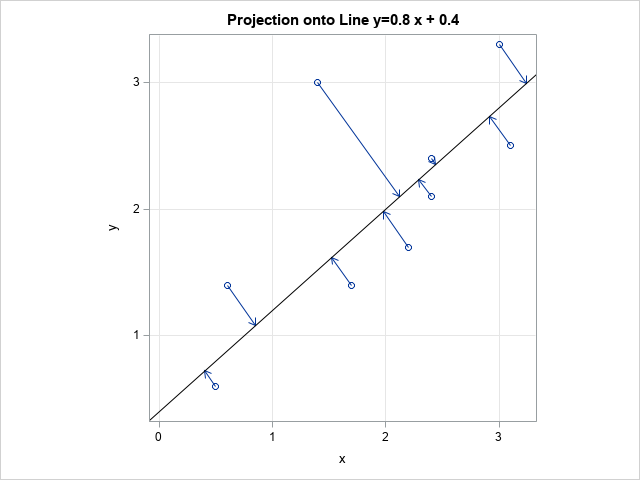A SAS programmer wanted to create a graph that illustrates how Deming regression differs from ordinary least squares regression. The main idea is shown in the panel of graphs below.

• The first graph shows the geometry of least squares regression when we regress Y onto X. ("Regress Y onto X" means "use values of X to predict Y.") The residuals for the model are displayed as vectors that show how the observations are projected onto the regression line. The projection is vertical when we regression Y onto X.
• The second graph shows the geometry when we regress X onto Y. The projection is horizontal.
• The third graph shows the perpendicular projection of both X and Y onto the identity line. This is the geometry of Deming regression.1. Given any line and any point in the plane, how do you find the location on the line that is closest to the point? This location is the perpendicular projection of the point onto the line.
2. How do you use the SGPLOT procedure in SAS to create the graphs that chow the projections of points onto lines?

The data for the examples are shown below:

```data Have; input x y @@; datalines; 0.5 0.6 0.6 1.4 1.4 3.0 1.7 1.4 2.2 1.7 2.4 2.1 2.4 2.4 3.0 3.3 3.1 2.5 ;```

### The projection of a point onto a line

Assume that you know the slope and intercept of a line: y = m*x + b. You can use calculus to show that the projection of the point (x0, y0) onto the line is the point (xL, yL) where
xL = (x + m*(y – b)) / (1 + m2) and yL = m * xL + b.

To derive this formula, you need to solve for the point on the line that minimizes the distance from (x0, y0) to the line. Let (x, m*x + b) be any point on the line. We want to find a value of x so that the distance from (x0, y0) to (x, m*x + b) is minimized. The solution that minimizes the distance also minimizes the squared distance, so define the squared-distance function
f(x) = (x - x0)2 + (m*x + b - y0)2.
To find the location of the minimum for this function, set the derivative equal to zero and solve for the value of x:

• f`(x) = 2(x - x0) + 2 m*(m*x + b - y0)
• Set f`(x)=0 and solve for x. The solution is the value xL = (x + m*(y – b)) / (1 + m2), which minimizes the distance from the point to the line.
• Plug xL into the formula for the line to find the corresponding vertical coordinate on the line: yL = m * xL + b.

You can use the previous formulas to write a simple SAS DATA step that projects each observation onto a specified line. (For convenience, I put the value of the slope (m) and intercept (b) into macro variables.) The following DATA step projects a set of points onto the line y = m*x + b. You can use PROC SGPLOT to create a scatter plot of the observations. Use the VECTOR statement to draw the projections of the points onto the line.

```/* projection onto general line of the form y = &m*x + &b */ %let b = 0.4; %let m = 0.8; data Want; set Have; xL = (x + &m *(y - &b)) / (1 + &m**2); yL = &m * xL + &b; run;   title "Projection onto Line y=&m x + &b"; proc sgplot data=Want aspect=1 noautolegend; scatter x=x y=y; vector x=xL y=yL / xorigin=x yorigin=y; /* use the NOARROWHEADS option to suppress the arrow heads */ lineparm x=0 y=&b slope=&m / lineattrs=(color=black); xaxis grid; yaxis grid; run;```You can get the graph for Deming regression by setting b=0 and m=1 in the previous formulas and program.

In summary, you can use that math you learned in high school to find the perpendicular projection of a point onto a line. You can then use the VECTOR statement in PROC SGPLOT in SAS to create a graph that illustrates the projection. Such a graph is useful for comparing different kinds of regressions, such as comparing least-squares and Deming regression.

The post Visualize residual projections for linear regression appeared first on The DO Loop.